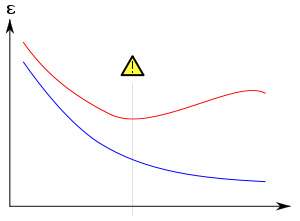# Overfitting

﻿
OverfittingNoisy (roughly linear) data is fitted to both linear and polynomial functions. Although the polynomial function passes through each data point, and the linear function through few, the linear version is a better fit. If the regression curves were used to extrapolate the data, the overfit would do worse.

In statistics, overfitting[clarification needed] occurs when a statistical model describes random error or noise instead of the underlying relationship. Overfitting generally occurs when a model is excessively complex, such as having too many parameters relative to the number of observations. A model which has been overfit will generally have poor predictive performance, as it can exaggerate minor fluctuations in the data.

The possibility of overfitting exists because the criterion used for training the model is not the same as the criterion used to judge the efficacy of a model. In particular, a model is typically trained by maximizing its performance on some set of training data. However, its efficacy is determined not by its performance on the training data but by its ability to perform well on unseen data. Overfitting occurs when a model begins to memorize training data rather than learning to generalize from trend. As an extreme example, if the number of parameters is the same as or greater than the number of observations, a simple model can learn to perfectly predict the training data simply by memorizing the training data in its entirety. Such a model will typically fail drastically on unseen data, as it has not learned to generalize at all.

The potential for overfitting depends not only on the number of parameters and data but also the conformability of the model structure with the data shape, and the magnitude of model error compared to the expected level of noise or error in the data.

Even when the fitted model does not have an excessive number of parameters, it is to be expected that the fitted relationship will appear to perform less well on a new data set than on the data set used for fitting. In particular, the value of the coefficient of determination will shrink relative to the original training data.

In order to avoid overfitting, it is necessary to use additional techniques (e.g. cross-validation, regularization, early stopping, pruning, Bayesian priors on parameters or model comparison), that can indicate when further training is not resulting in better generalization. The basis of some techniques is either (1) to explicitly penalize overly complex models, or (2) to test the model's ability to generalize by evaluating its performance on a set of data not used for training, which is assumed to approximate the typical unseen data that a model will encounter.

## Machine learningOverfitting/Overtraining in supervised learning (e.g. neural network). Training error is shown in blue, validation error in red, both as a function of the number of training cycles. If the validation error increases(positive slope) while the training error steadily decreases(negative slope) then a situation of overfitting may have occurred. The best predictive and fitted model would be where the validation error has its global minimum.

The concept of overfitting is important in machine learning. Usually a learning algorithm is trained using some set of training examples, i.e. exemplary situations for which the desired output is known. The learner is assumed to reach a state where it will also be able to predict the correct output for other examples, thus generalizing to situations not presented during training (based on its inductive bias). However, especially in cases where learning was performed too long or where training examples are rare, the learner may adjust to very specific random features of the training data, that have no causal relation to the target function. In this process of overfitting, the performance on the training examples still increases while the performance on unseen data becomes worse.

As a simple example, consider a database of retail purchases that includes the item bought, the purchaser, and the date and time of purchase. It's easy to construct a model that will fit the training set perfectly by using the date and time of purchase to predict the other attributes; but this model will not generalize at all to new data, because those past times will never occur again.

Generally, a learning algorithm is said to overfit relative to a simpler one if it is more accurate in fitting known data (hindsight) but less accurate in predicting new data (foresight). One can intuitively understand overfitting from the fact that information from all past experience can be divided into two groups: information that is relevant for the future and irrelevant information (“noise”). Everything else being equal, the more difficult a criterion is to predict (i.e., the higher its uncertainty), the more noise exists in past information that need to be ignored. The problem is determining which part to ignore. A learning algorithm that can reduce the chance of fitting noise is called robust.